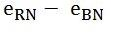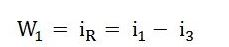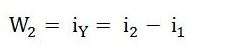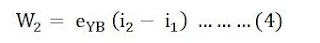# Two Wattmeter Method For Power Measurement

Two-watt meter method is used for measuring power in 3 phase 3 – wire star or delta connected systems either with the balanced or unbalanced load. Let us discuss the two wattmeter method for 3 phase power measurement with star and delta connection in detail.

## Measurement of power by the two wattmeter method in star connection:

In this method, we use two-watt meters and the current coils of these watt meters are connected in series with two lines and potential coils are connected across these two lines and the load is connected in the star as shown in the following figure.From figure
The instantaneous current flowing through the watt meter W1 is Ir
The voltage across the potential coil of watt meter W1 isInstantaneous power measured by the wattmeter, W1 is given by,The instantaneous current flowing through the watt meter W2 is Iy
The voltage across the potential coil of watt meter W2 isInstantaneous power measured by the wattmeter, W2 is given byThe total 3 phase power will be the sum of power measured by watt meters W1 and W2
Adding equations 1 and 2 we get following results.Where P is the total 3 phase power consumed by the load.

## Measurement of Power by the two wattmeter method in delta connection:

In this method also we use two-watt meters and the current coils of these watt meters are connected in series with two lines and potential coils are connected across these two lines and the load is connected in delta as shown in the following figure.The instantaneous current flowing through the watt meter W1 is,The voltage across the potential coil of watt meter W1 is Erb
Instantaneous power measured by the wattmeter, W1 is given by,The instantaneous current flowing through the watt meter W2 is,The voltage across the potential coil of watt meter W2 is Eyb
Instantaneous power measured by the wattmeter, W2 is given by,The total 3 phase power will be the sum of power measured by watt meters W1 and W2
Adding equations 3 and 4 we get following results.Where P is the total 3 phase power consumed by the load.
Note: The power measured by watt meter is average power because of the inertia of their moving system.
In this post, we have discussed measure element of three-phase power using the two wattmeter method